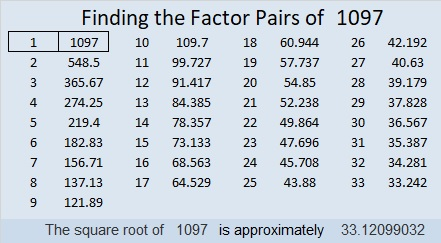# 1097 and Level 3

72 and 27 are mirror images of each other. What is the largest number that will divide evenly into both of them? Put the answer to that question under the x, and you will have completed the first step in solving this multiplication table puzzle.Print the puzzles or type the solution in this excel file: 12 factors 1095-1101

Here’s a little bit more about the number 1097:

• 1097 is a prime number.
• Prime factorization: 1097 is prime.
• The exponent of prime number 1097 is 1. Adding 1 to that exponent we get (1 + 1) = 2. Therefore 1097 has exactly 2 factors.
• Factors of 1097: 1, 1097
• Factor pairs: 1097 = 1 × 1097
• 1097 has no square factors that allow its square root to be simplified. √1097 ≈ 33.12099

How do we know that 1097 is a prime number? If 1097 were not a prime number, then it would be divisible by at least one prime number less than or equal to √1097 ≈ 33.1. Since 1097 cannot be divided evenly by 2, 3, 5, 7, 11, 13, 17, 19, 23, 29 or 31, we know that 1097 is a prime number.1097 is the final prime number in the prime triplet, 1091-1093-1097.

1097 is the sum of two squares:
29² + 16² = 1097

1097 is the hypotenuse of a primitive Pythagorean triple:
585-928-1097 calculated from 29² – 16², 2(29)(16), 29² + 16²

Here’s another way we know that 1097 is a prime number: Since its last two digits divided by 4 leave a remainder of 1, and 29² + 16² = 1097 with 29 and 16 having no common prime factors, 1097 will be prime unless it is divisible by a prime number Pythagorean triple hypotenuse less than or equal to √1097 ≈ 33.1. Since 1097 is not divisible by 5, 13, 17, or 29, we know that 1097 is a prime number.

This site uses Akismet to reduce spam. Learn how your comment data is processed.